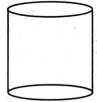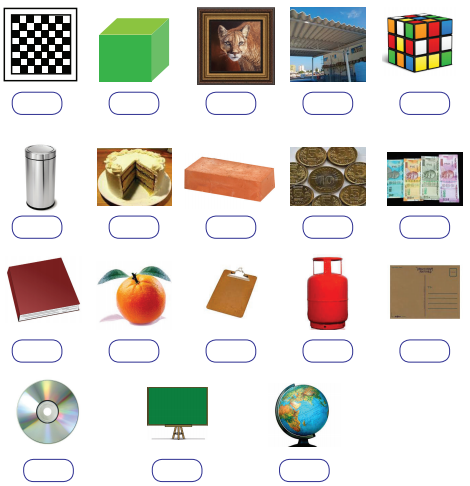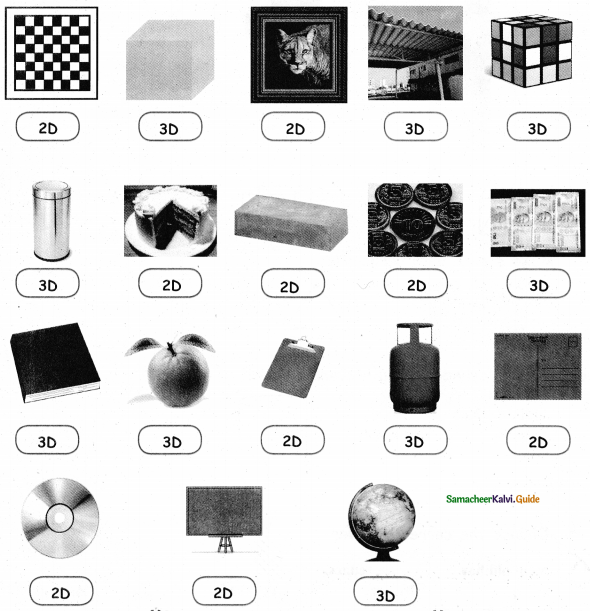Students can download 4th Maths Term 1 Chapter 1 Geometry InText Questions and Answers, Notes, Samacheer Kalvi 4th Maths Guide Pdf helps you to revise the complete Tamilnadu State Board New Syllabus, helps students complete homework assignments and to score high marks in board exams.

## Tamilnadu Samacheer Kalvi 4th Maths Solutions Term 1 Chapter 1 Geometry InText Questions

Activity (Text Book Page No. 8)

Measure the radius and diameter of the following circles.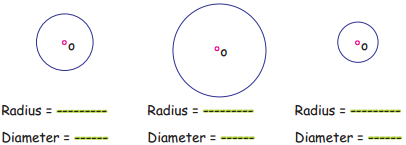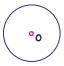Diameter = 2 cm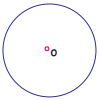Diameter = 3.4 cm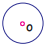Diameter = 1.4 cm

Activity (Text Book Page No. 9)

Measure the sides arid identify the names of different objects and find the differences among them and fill the table given below.
(a) Chessboard
(b) Postcard
(c) Window
(d) Paper
(e) Newspaper
(f) Maths Kit box.
(g) Kite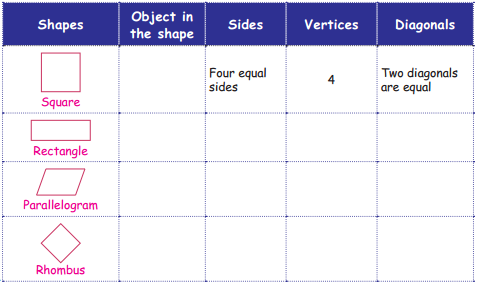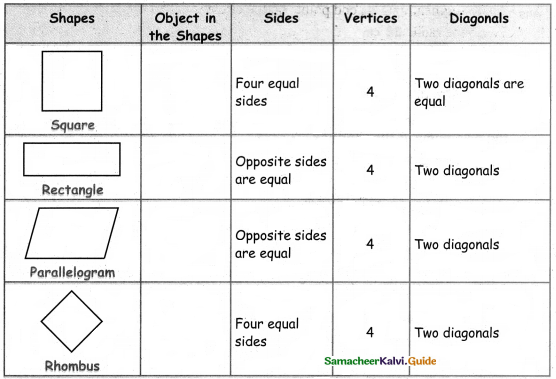Activity (Text Book Page No. 12)

Arrange the tangram pieces to from pictures.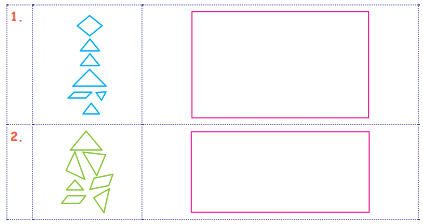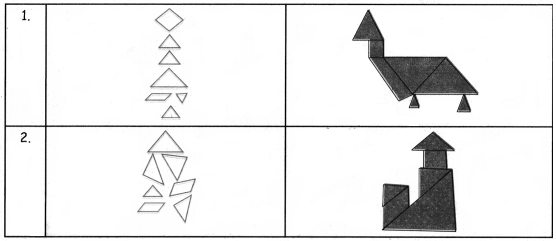Activity (Text Book Page No. 14)

Which tile will you choose to fill the space given below and find how many tiles are needed to fill the given space.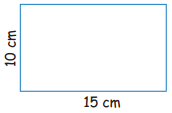(a)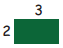(b)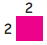(c)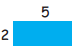(a)Perimeter = 2 + 3 + 2 + 3 = 10 cm
No of tiles needed = 5Activity (Text Book Page No. 14 & 15)

Fill the table given below by fixing the appropriate tile in the space given below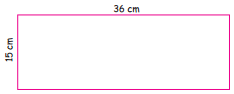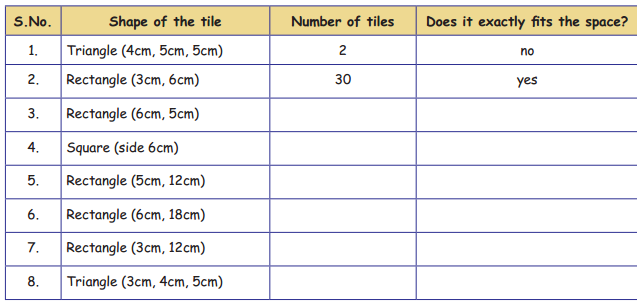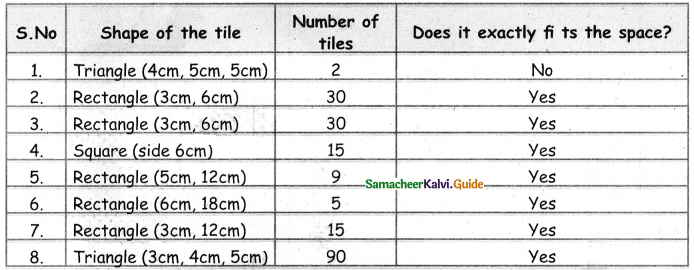Activity (Text Book Page No. 16)

a. Form the cube by folding the nets given below.
Question 1.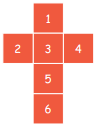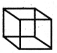Question 2.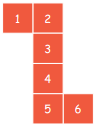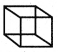Question 3.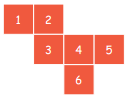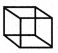b. Use these nets to form cuboids.

Question 1.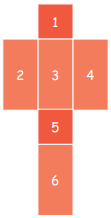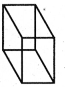Question 2.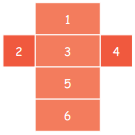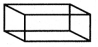c. Make a cone with semicircle.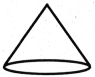d. Make a cylinder using rectangle sheet.